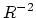Next: Notions of relativistic dynamics Previous: Dynamics of the SRT   Contents

# Introduction

In the previous Chapters we have proved the inconsistency of kinematic concepts of SRT, the groundlessness of GRT, the invalidity of relativistic interpretations of some key experiments (after this we could regard the relativity theory as some mnemonic rule, but it is rather awkward and unwise). Although this is quite enough in order to seek interpretations of observed phenomena other than relativistic ones, nevertheless, the present Chapter 4 supplements to the aforementioned systematic criticism of the relativity theory. The fact is, that all textbooks (beginning with school ones) incite in favour of so-called progress which is based on achievements of modern science. The relativity theory is boosted as one of its foundations, and for some reason atomic bombs and accelerators are mentioned in this case. However, even here situation is not unclouded (though theorists fanatically believe that their written "flourishs" bear a direct relation to the Reality): no one accelerator reachs the rated capacity on "ideal" theoretical calculations. Phenomenological formulae and "fitting" parameters and factors are used in the majority of cases for practical courses and engineering calculations. The main purpose of this Chapter 4 is to demonstrate that even in a seemingly unique practical SRT section, namely, in the relativistic dynamics, there exist numerous questions, compelling one to doubt in the validity of relativistic ideas and in interpreting their results.

It is well known a philosophical statement (legibly applicable to SRT): "we can see that thing in the experiment we want to see there". Such an attitude is prepared and the situation is aggravated by the theorists, who are "stewed in their own juice" and ready to see in every experiment only confirmation to their tricks with mathematical symbols (although the author belongs to theorists as well). The existing uncertainties of the theory (carefully masked in SRT) allow the theorists to vary interpretation of experiments within considerable limits. And, afterwards, the incompleteness of experiments is masked "in a proper manner" by statistical "adjustment" of the data (data "truncation" under the desirable result).

In deriving the equations of motion of an electric charge and the field equations in theoretical physics' courses an attempt is made to cause an illusion of an "unequivocal idyll". But in such a case the Maxwell equations would be the equations of any fields, and all forces would be of Lorentz type and would have the form of Coulomb's law in a static case. Such an alternative of the general relativity theory (GRT) can be discussed (with some supplementation and modifications) for the gravitational field. However, the situation is different in the general case: for example, the nuclear forces are not proportional to. There exist many counterexamples of various fields and forces. Therefore, the theoretical physics (including the SRT approach) cannot determine all existing phenomena proceeding from their own principles only. This is an exclusive prerogative of the experiment. (Besides, the experimenter should be principally prepared to the fact that any theory can occur to be inaccurate or even wrong).

Also surprising is the apologetic advertising of SRT. For example, the pathos's assertion of , that "the relationship between the mass and energy underlies the entire nuclear power engineering" is groundless both in the historical and in the practical respect. This relationship bears no relation either to discovery of elementary particles and radioactivity, or to studying the spontaneous and forced decaying of uranium nuclei, or to determining the stability of nuclei, or to finding possible channels of nuclear reactions and possibility of practical choice between them, or to the isotope separation technology, or to practical utilization of released energy, etc. Thus, this relationship bears no relation to any key stage in development of nuclear power engineering. And (as paradoxical it may seem) this relationship bears no relation even to determining the released energy in any particular well-known reaction. This is because all things have historically happened just in the opposite order: at first, some reaction has been found, which was detected from the energy release exactly. And then the calculational functions - the combinations from mathematical symbols - can be derived by various methods. As a rule, it is technically impossible to determine the mass variation in a nuclear reaction directly. Even if one uses doubtful theoretical interpretations, the attempt to determine the mass variation will occur to be a rather rough and costly pleasure. Thus, the relationship between the mass and energy plays, in the practical respect, a part of scholar mathematical exercises for reverse substitutions, since desirable results can always be "derived" from the calculated data, which were tabulated post factum.Next: Notions of relativistic dynamics Previous: Dynamics of the SRT   Contents
Sergey N. Arteha